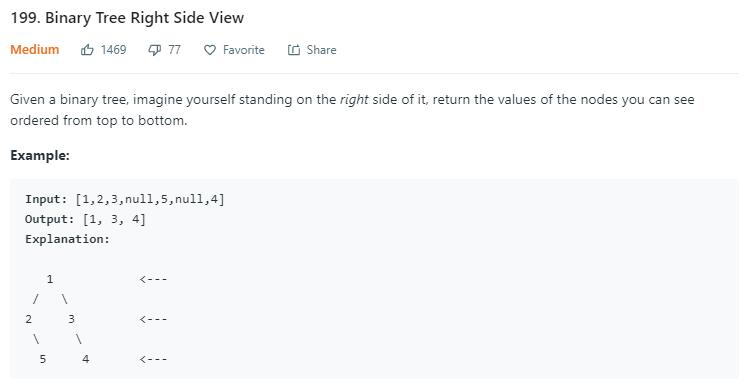# 题目描述（中等难度）# 解法一

public List<Integer> rightSideView(TreeNode root) {
if (root == null)
return res;
queue.offer(root);
while (!queue.isEmpty()) {
int levelNum = queue.size(); // 当前层元素的个数
for (int i = 0; i < levelNum; i++) {
TreeNode curNode = queue.poll();
//只保存当前层的最后一个元素
if (i == levelNum - 1) {
}
if (curNode.left != null) {
queue.offer(curNode.left);
}
if (curNode.right != null) {
queue.offer(curNode.right);
}

}
}
return res;
}


# 解法二

public List<Integer> rightSideView(TreeNode root) {
rightSideViewHelper(root, 0, res);
return res;
}

private void rightSideViewHelper(TreeNode root, int level, List<Integer> res) {
if (root == null) {
return;
}
//res.size() 的值理解成当前在等待的层级数
//res.size() == 0, 在等待 level = 0 的第一个数
//res.size() == 1, 在等待 level = 1 的第一个数
//res.size() == 2, 在等待 level = 2 的第一个数
if (level == res.size()) {